Next: How can one remove Up: Convex Polyhedron Previous: How hard is it   Contents

## Is there an efficient way of determining whether a given point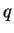is in the convex hull of a given finite set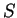of points in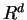?

Yes. However, we need to be careful.

First we give a method that we do not recommend but many people use. This method computes an inequality representation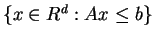of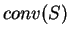where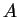is some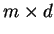matrix and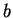is a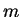-vector. This is called the convex hull computation 2.10. Once the system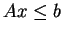is computed, it is easy to check whether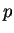satisfies the system or not.

In most cases, this method is too expensive, since the convex hull computation is very hard in general and impossible for large data. In fact, the number of inequalities in such a systemis often exponential in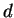and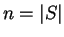. (This method might be of practical interests when we need to remove lots of redundant points in clouds of points in small dimensions, see 2.20.)

A standard method to check whetheris inuses linear programming (LP) technique 4. An LP problem to be formulated for the question is the following. Let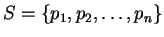.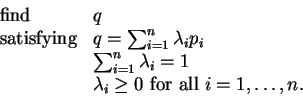(3)

This problem has no objective function and such a problem is often called a linear feasibility problem. Although it might look simpler problem to solve, it is polynomially equivalent to the general LP. In fact, it is usually a good idea to set up an equivalent LP to solve it. More specifically, the problem (3) has a solution if and only if the following has no solution: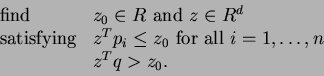(4)

Geometrically, the meaning of this problem is simple. If it admits a solution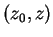, then the set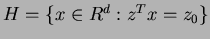is a hyperplane inseparating the polytopefrom the inquiry point. Thus the existence of the separation means the nonredundancy. Now, to actually solve the problem (4), we set up the LP: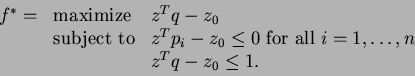(5)

The last inequality is artificially added so that the LP has a bounded solution. It is easy to see that the pointis non-redundant if and only if the optimal value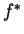of the LP (5) is (strictly) positive.Next: How can one remove Up: Convex Polyhedron Previous: How hard is it   Contents
Komei Fukuda 2004-08-26